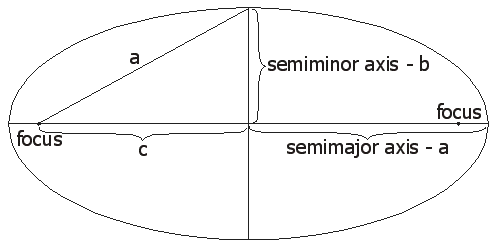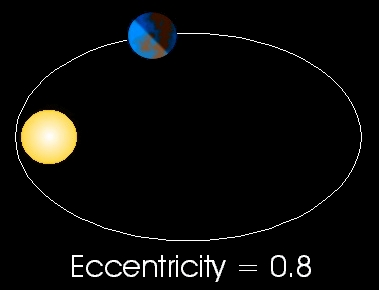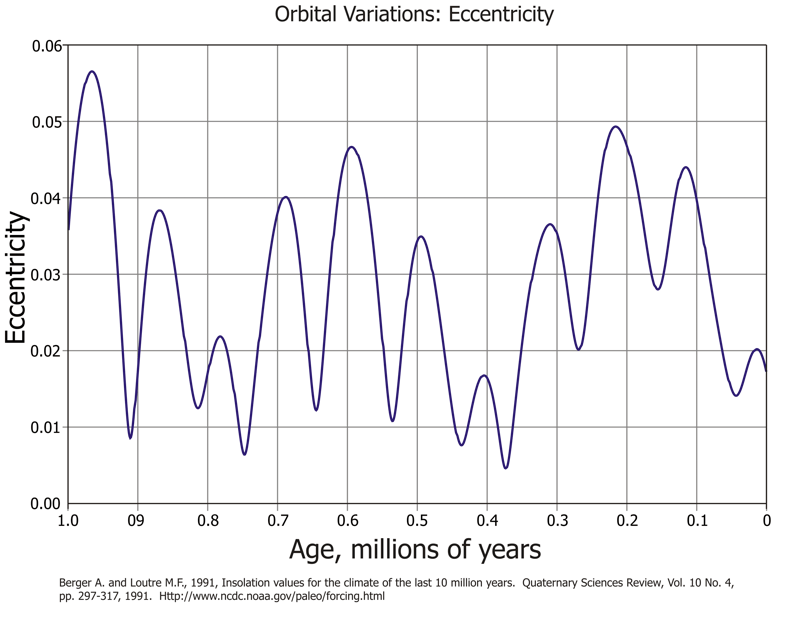# Orbital Eccentricity

### Elliptical Orbits

The orbits of the planets are ellipses with the Sun at one focus.Geometry of an Ellipse

While the orbits of the planets are elliptical, it should be noted that the orbits of most of the planets are nearly circular. Geometrically a circle is just a special case ellipse in which the semimajor and semiminor axes are equal in length. For example, Earth's nearest approach to the Sun is 147 million kilometers. Earth's maximum distance from the Sun is 152 million kilometers. This is a difference of 5 million kilometer - a large distance on Earth but a relatively small distance when compared to the total distance to the Sun. Comets show the greatest eccentricity of the objects orbiting the Sun (very long semimajor axes with very short semiminor axes).

### Eccentricity

Eccentricity is a measure of an ellipse's circularity. The equation e = c/a allows one to calculate the eccentricity, where e is the eccentricity, c is the distance from the center of an ellipse to a focus, and a is the semimajor axis length. The value for c is easily determined using the equation:

c2 = a2 - b2

Eccentricity, e, will vary between 0 and 1. An ellipse where e equals zero is a circle, and an ellipse where e equals one is a line. Another way of thinking about this is the closer e is to 1, the more elongated the ellipse will be.

The series of diagrams below show examples of different orbital eccentricities. The star and planet sizes have been greatly exaggerated. In each case the semimajor axis is the same.### Earth's Eccentricity

The Earth's orbital eccentricity varies from a maximum to minimum eccentricity over a period of approximately 92,000 years. The maximum eccentricity for the Earth is 0.057, while 0.005 is the minimum. Currently, 0.0167 is the Earth's eccentricity. Because of these very small eccentricities, any diagrams drawn to scale of the Earth's orbit would all look circular. The table below shows the actual distances involved. Due to the astromonical distances involved, note how even when the Earth's eccentricity is at a minimum, the difference between the Earth's closet and farthest approach to the sun is just under 1.5 million kilometers. The diagram below the table shows how Earth's eccentricity has varied over the past 1 million years.

Earth's Orbital Eccentricity
Eccentricity
e
Semimajor
axis (km)
Semiminor
axis (km)
Minimum
Distance
to Sun (km)
Maximum
Distance
to Sun (km)
Maximum e 0.057 149,597,900 149,354,680 141,070,819 158,124,980
Current e 0.0167 149,597,900 149,577,046 147,099,615 152,096,185
Minimum e 0.005 149,597,900 149,596,030 148,849,910 150,345,890